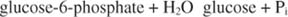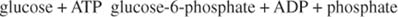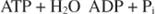## Free‐Energy Calculations

Biochemical free energies are usually given as standard free energies of hydrolysis. For example, the hydrolysis of glucose‐6‐phosphate:has ΔG° = ‐4.0 kcal/mole (‐16.5 kJ/mole) under standard conditions. Therefore, the opposite reaction, the phosphorylation of glucose, is unfavored. However, the phosphorylation of glucose occurs readily in the cell, catalyzed by the enzyme hexokinase:The other half of the phosphorylation reaction is the hydrolysis of ATP to yield ADP and inorganic phosphate (P i):under standard conditions has ΔG° = ‐7.3 kcal/mole (‐31 kJ/mole).

The standard free energy change of the reaction can be determined by adding the two free energies of reaction:Note that the reaction as written is unfavored; its free energy change is positive. Another way of stating this is that the reaction is endergonic, that is, the reaction involves a gain of free energy.

For the exergonic hydrolysis of ATP (the reaction involves a loss of free energy):The two reactions are summed:This is a simple example of energetic coupling, where an unfavorable reaction is driven by a favorable one, as shown in Figure 1 .Figure 1

Coupling doesn't occur all by itself. In this example, if this experiment were set up so that the ATP would have to be hydrolyzed in one tube and the glucose phosphorylated in another, no coupling would be possible. Coupling can occur only when the partial reactions are part of a larger system. In this example, coupling occurs because both partial reactions are carried out by the enzyme hexokinase. In other cases, coupling can involve membrane transport, transfer of electrons by a common intermediate, or other processes. Another way of stating this principle is that coupled reactions must have some component in common.